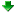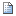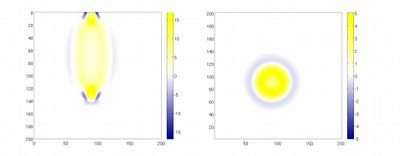# FD3S(AD)

FD3S(AD) is an extension of FD3S (see above) that allows to compute the derivative of an objective functional defined on a recorded seismic wavefield with respect to the model parameters. The computation of the derivative is based on the adjoint method.

Authors: Andreas Fichtner Wave propagation Research Fortran 90/95 or later Planetary Scale Distributed Memory (e.g., MPI) 3D Spherical Finite differences Linux Elastic anisotropic Free surface (planar), Absorbing boundaries Rectangular, regular Numerical 5887

Additional resources
•All Releases
•Change logs

## Project Description

FD3S(AD) is an extension of FD3S (see above) that allows to compute the derivative of an objective functional defined on a recorded seismic wavefield with respect to the model parameters. Typical parameters are seismic wave speeds, elastic constants or density. The choice of the objective functional is made through the adequate definition of adjoint sources.

Since the computation of the derivatives is based on the adjoint method, three wavefield simulations are needed:

1) Computation of the forward wavefield: The forward wavefield, recorded at specified receiver locations, is computed with a slightly modified version of FD3S. In the course of the simulation the wavefield at the absorbing boundaries is stored in order to allow the reverse time stepping.

2) Computation of the adjoint field: The adjoint wave field is set off by the adjoint sources which have to be defined by the user on the basis of his or her choice of the objective functional. The adjoint field also satisfies the elastic wave equation. However it is anti-causal, meaning that it propagates from the future into the past. This implies that the simulation of the adjoint field has to be done backward in time.

3) Since the computation of the objective function derivative requires knowledge of both the adjoint wavefield and the forward wavefield at any given point in time, the adjoint field is modelled simultaneously with the backward propagating forward field. Both fields are then combined to give the objective function derivative.

All calculations are done without dissipation. If dissipation were included, the entire forward field would have to be stored at every time step. This however clearly exceeds usual storage capacities.

## Screenshot